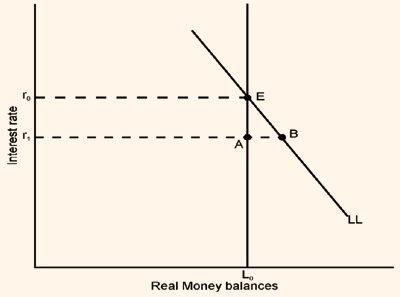Equilibrium in money markets, Macroeconomics

Assignment Help:

Equilibrium in Money Markets

Having dealt with the forces that determine the supply of money and demand for money, let us combine supply of and demand for money to determine equilibrium in money markets.

The money markets will be in equilibrium when the quantity of real balances demanded equals the quantity supplied.

The real money supply is the nominal money supply divided by the price level. The central bank controls the nominal money supply. The central bank could be assumed to control the real money supply if, for theoretical purposes, we assume the prices of goods to be fixed. The nominal money supply i.e. currency with public and deposit money with public equals the monetary base or high powered money (i.e. currency plus commercial banks' deposits at the bank) multiplied by the money multiplier.

Demand for money is the demand for real money balances. The quantity of real money demanded increases with the level of real income but decreases with the level of nominal interest rates.

Figure 7.1 shows the demand curve LL for real money balances for a given level of real income.

Figure 7.1The higher the interest rate and the opportunity cost of holding money, the lower the quantity of real money balances demanded. With a given price level, the central bank controls the quantity of nominal money and real money. The supply curve is vertical at this quantity of real money L0. Equilibrium is at the point E. At the interest rate r0 the quantity of real money that people wish to hold just equals the outstanding stock L0.

Suppose the interest rate is r1, lower than the equilibrium level r0. There is an excess demand for money given by the distance AB in Figure 7.1. How does this excess demand for money bid the interest rate up from r1 to r0 to restore equilibrium? The answer to this question is rather subtle.

A market for money would involve buying and selling rupees with other rupees, which makes no sense.

The other market of relevance to Figure 7.1 is the market for bonds. In saying that the interest rate is the opportunity cost of holding money, we are saying that people who do not hold money will hold bonds instead.

Thus, the stock of real wealth W is equal to the total outstanding stock or supply of real money L0 and real bonds B0. People have to decide how they wish to divide up their total wealth W between desired real bond holdings BD and desired real money holdings LD. Whatever factors determine this division, it must be true that

L0 + B0 = W = LD + B                                                       ...(7.14)

The total supply of real assets determines the wealth to be divided between real money and real bonds. And people cannot plan to divide up wealth they do not have. Since the left-hand side of equation (7.14) must equal the right-hand side, it follows that

B0 - BD = LD - L0                                                                ...(7.15)

An excess demand for money must be exactly matched by an excess supply of bonds. Otherwise people would be planning to hold more wealth than they actually possess.

This insight allows us to explain how an excess demand for money at the interest rate r1 in Figure 7.1 sets in motion forces that will bid up the interest rate to its equilibrium level r0. With excess demand for money, there is an excess supply of bonds. To induce people to hold more bonds, suppliers of bonds must offer a higher interest rate. As the interest rate rises, people switch out of money and into bonds. The higher interest rate reduces both the excess supply of bonds and the excess demand for money. At the interest rate r0 the supply and demand for money are equal. Since the excess demand for money is zero, the excess supply of bonds is also zero. The money market is in equilibrium only when the bond market is also in equilibrium. People wish to divide their wealth in precisely the ratio of the relative supplies of money and bonds.

Market struture, barriers to entry?

barriers to entry?

Difference between a normal good and an inferior good, Price/Feeder Quantit...

Price/Feeder Quantity Demanded Quantity Supplied \$300 500 1800 270 600 1700 240 700 1600 210 800 1500 180 1000 1400 150 1100 1300 120 1200 1200 90 1300 1100 60 1400 1000 30 1500 90

what is gdp

Find real interest rate and nominal interest rate, Assume that an economy's...

Assume that an economy's GDP Y=5000. Also assume that the government runs a deficit where tax revenue T=1000 and government expendituresG= 1500. The consumption function is represe

The short-run macroeconomic equilibrium, The figure below defines an econom...

The figure below defines an economy's aggregate demand curve and its short-runand long-run aggregate supply curves (labelled AD, SRAS, and LRAS, respectively). practically,the econ

#title.production, the uses of production function

the uses of production function

Impact will high and variable rates of inflation, What impact will high and...

What impact will high and variable rates of inflation have on the economy? How will they influence the risk accompanying long-term contracts and related business decisions?

Describe short-run equilibrium price-demand, The aim of this task is to exp...

The aim of this task is to explore the effects of a supply shock on a firm and thereby on the industry. Suppose that war breaks out in the Middle East, where a considerable portion

Nominal vs. Real wage, Do we get paid nominal or real wage?

Do we get paid nominal or real wage?